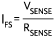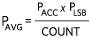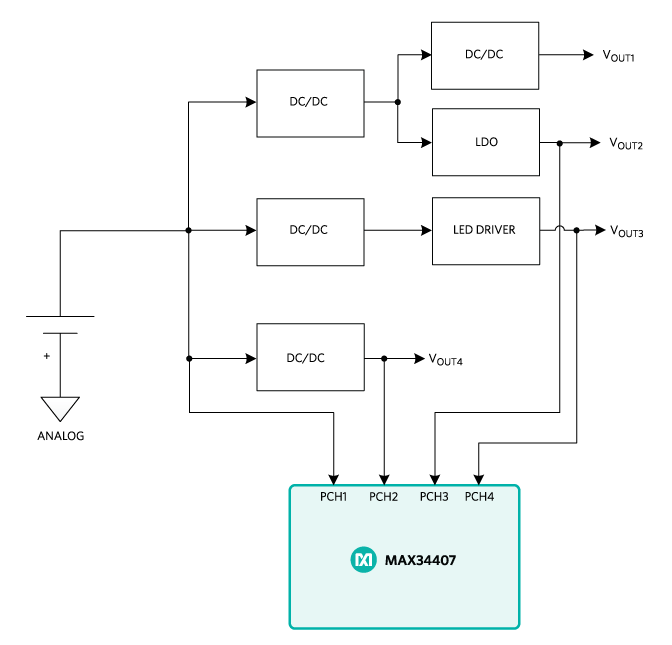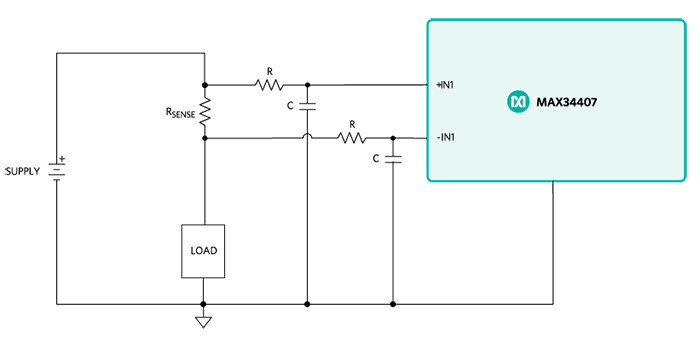# How to Perform Real-Time Power Measurements with a Power Accumulator

### Abstract

For many applications that need average power measurement, power accumulators are a good solution. Consider real-time measurement of switching converter efficiency, which allows converter efficiency evaluation over time and under varying operating conditions. By extending this approach to multiple rails, you can monitor power management integrated circuits (PMICs) in battery-powered, high-density systems. Power accumulation also facilitates rapid prototyping by monitoring various system rails during development. The logged data can be leveraged for continuous system design improvement. This application note discusses various applications where a power accumulator can be invaluable in delivering critical, real-time power measurements.

### Introduction

Measuring the power consumed by critical system rails in an electrical system provides telemetry of vital system functions. Real-time power consumption data enables a system to perform dynamic optimization to prolong battery life. Power monitors, which sample the voltage and current of a rail to generate an instantaneous power measurement, are often used in systems that constantly monitor the peak power of a system rail. However, average power calls for greater computation. In these situations, instantaneous power readings must be accumulated over a specified time interval in order to generate an average power measurement. Power monitors would need additional processing to perform this kind of measurement, a difficult endeavor for systems with limited processing capacity. This is why traditional power monitors are not ideal for average power measurements. Power accumulators are a better option here because they have the accumulation feature integrated to provide a system host with average power readings.

### How Power Accumulators Work

To obtain an accumulated power value, power accumulators measure voltage and current samples, multiply them for a power reading, and add multiple readings. The chip stores the accumulated power and sample count information. Figure 1, which depicts a MAX34407 block diagram, provides an example of this process.Figure 1. MAX34407 power accumulator block diagram.

Using a multiplexer, current-sense amplifier, and 12-bit ADC, the MAX34407 automatically collects current and voltage samples from four channels. To generate a 28-bit power value for each sample pair, these voltage and current samples are multiplied on-chip. The power values are added and stored in a 48-bit register, and the count of instantaneous power samples accumulated is stored in a 24-bit register. The MAX34407 doesn’t need an external sample clock, so, with an automatic sequencer and integrated oscillator, the chip is fully autonomous.

With an I2C/SMBus command from the host, the device transfers the accumulated power samples and count to a set of registers available to the host. The host can then retrieve data at any time interval, and the transfer occurs without missing a sample. In normal mode, the MAX34407 samples at a rate of 1024sps. Since the accumulation depth is 48 bits while individual samples are 28 bits, 220 samples can be stored before the host controller needs to read back the data:After the host has received power accumulation and count data, a basic computation reveals the average load power. Without the burden of accumulating power data, the host processor can focus on managing more important tasks. The power accumulation data is converted by the host processor using a scaling factor as determined with the following constants and equations. PACC is the accumulated power value and COUNT is the total number of power samples:

MAX34407-specific constants:

• Voltage-sense full scale, VFS = 16V
• Current-sense full scale, VSENSE = 100mV
• Power-accumulated word length, 28 bits
Scaling factor:
• Full-scale current,• Power-scale correction, PSCALE = VFS × IFS
• Least significant power bit,Average power calculation:
•The above provides an example using the MAX34407 to convert the accumulated ADC count to average power. Since power accumulators have different features and operation, be sure to consider the specific operation of each power accumulator.

### Taking Efficiency Measurements with a Single IC

For any power converter, efficiency is one of the most important specifications. After a design has been implemented, the designer or end-user wouldn’t have access to real-time converter efficiency measurements. These measurements vary over time and operating conditions, and can also be influenced by factors like component drift, load conditions, temperature, and humidity. A power accumulator with multiple rails provides a single IC approach for taking efficiency measurements and, overall, monitoring a converter’s efficiency in real time.

Since the MAX34407 accumulates power on multiple rails, you can measure efficiency by accumulating power at the input (PIN) and output (POUT) of a converter. The result of these two measurements can be sent to a microprocessor for efficiency computation using this formula:In Figure 2 you can see a typical circuit for measuring efficiency of the MAXM17504 step-down converter using the MAX34407 power accumulator. An example application for this circuit is a 12V solar panel system where the primary converter typically incorporates a maximum power point tracking (MPPT) algorithm. Since real-time efficiency measurements provide feedback of MPPT algorithm performance, the system or designer can optimize the MPPT algorithm based on in-circuit measurements. MAXM17504 input voltage range is 12V ± 10%, which fits into MAX34407 common-mode voltage range of +2.7V to +15V. The full-scale range of the current-sense amplifier is 100mV, so a 50mΩ resistor was used on the input for 2A maximum input current and a 25mΩ resistor was used on the output for 4A maximum output current.Figure 2. Efficiency measurement application circuit.

Figure 3 shows an alternative way to measure efficiency by using four benchtop digital multimeters (DMMs). By measuring input current, output current, input voltage, and output voltage, the 4-DMM method provides a highly accurate efficiency measurement. This approach can be used as a baseline measurement for comparison with the efficiency measurement obtained from the power accumulator IC. See Figure 4 for an efficiency comparison between MAX34407 and the 4-DMM efficiency measurement method.Figure 3. Efficiency measurement circuit using 4 DMMs.Figure 4. MAX34407 efficiency measurement vs. 4-DMM method.

There is a notable offset in the two methods, and this stems from the power dissipated in the output current-sense resistor. Since MAX34407 measures the common-mode load voltage from the lower voltage terminal of the sense resistor, this value is included in the input power measurement rather than in the output power measurement. You can compensate measured output power by adding the calculated sense resistor power dissipation, which yields the total output power. Below, you can see the equations used to compensate the efficiency measurements. POUT and VOUT are measured by MAX34407 and RSENSE is a known value. Figure 5 displays the results of the compensated efficiency measurement.Figure 5. MAX34407 compensated efficiency measurement vs. 4-DMM method.

The results of Figure 5 demonstrate a maximum difference of -0.5% between the 4-DMM and MAX34407 efficiency measurements. What this reveals is that the MAX34407 can be used to accurately measure in-system efficiency of a switching converter with proper output power compensation. Note that the compensation technique works best for converters with a ripple voltage of 1% or less.

### How Much Power Are Your PMICs Consuming?

Monitoring PMIC voltage rails and determining system performance based on power consumption is important for extending battery life. To determine which system blocks consume the most power, you can utilize power accumulation diagnostics. The resulting data would allow the microcontroller to adjust functionality when a system block is using a significant portion of remaining battery capacity.

Figure 6 depicts a typical PMIC power monitoring application circuit using the MAX34407 power accumulator and MAX77650 PMIC. The MAX77650 contains a single-inductor multiple-output (SIMO) converter, which uses a single inductor with three integrated buck-boost outputs. This PMIC contains an analog multiplexer (AMUX), which can provide battery (BATT) or charging (CHGIN) information such as voltage or current. For an easy way to measure additional PMIC rails and also provide feedback to the central processor, you can add a power accumulator like MAX34407.Figure 6. PMIC power monitoring.

### Keeping an Eye on the Board

For systems with high-power requirements, the limited power capabilities of PMICs are not ideal. In these systems, multiple converters co-exist to supply critical system loads. Monitoring accumulated power can help you manage system loads based on available source power and, thus, optimize system efficiency. A typical power system is shown in Figure 7, where the MAX34407 is used to monitor critical system rails. This includes the input voltage rail to monitor the input power to the system. Furthermore, rails that consume the greatest power are monitored to determine system efficiency and to protect high-power outputs. Note that these converters can be remotely located, which would necessitate extensive routing to MAX34407. To ensure that switching noise does not impact power measurements, the MAX34407 must be properly routed. (See the Considerations for the Layout and Filtering Phases section of this application note for more details about layout considerations.)Figure 7. Board/system power monitoring.

### Monitoring Power When Prototyping

Fast development time continues to be essential in today’s competitive market. That is why solutions that are quick and easy to implement are becoming more desirable. Many developers use microcontroller platforms such as ARM® mbed®, Arduino™, and Raspberry Pi® for rapid prototyping. Through add-on boards, these hardware platforms can be easily upgraded. Plus, these daughterboards bring hardware functions that cannot be performed using the hardware on the platform board itself. Power accumulation is a function that can be used to monitor a prototype’s performance with quick implementation.

Using a power accumulator add-on board, you can perform power measurements of any system rail during the project development phase. This provides an easy way to measure the efficiency of your software, sensors or converters, as well as the general power consumption of any system block. If you need power measurements of individual modules, you can place sense resistors and terminal blocks d onto the add-on board. Alternatively, you can also route in-system sense resistors to a power accumulator add-on board instead of using the on-board sense resistors. Regardless of the application type, using a power accumulator provides valuable data during the design phase.

### Considerations for the Layout and Filtering Phases

In any instrumentation application, the layout phase is crucial when it comes to minimizing measurement error. Power monitoring does call for component placement near switching converters; however, switching interference induces error on power measurement. That is why it is very important to reduce coupled noise via proper routing. When noise is inevitable, you can place filters at the power monitor inputs to filter either common-mode noise or differential-mode noise. In an example of proper kelvin sense connections placed across the current-sense resistor, the two current-sense lines would be of equal length and would be routed in parallel to each other with minimal spacing in between.Figure 8. Kelvin sense connections.

The MAX34407 operates by taking high-side measurements, making it prone to common-mode noise. As a result, if the system exhibits excessive common-mode noise, additional filters might be needed. To filter unwanted noise, RC filters are placed in series with the current-sense input. In Figure 9, you can see a common-mode filter configuration using two identical RC filters on each current-sense line. This effectively forms a low-pass filter for common-mode signals. Since filter resistors are placed in series with the current-sense path, these resistors must be well matched to prevent offset in the power measurement. Because of this, the filter resistors used should have 1% or less tolerance. In addition, to avoid loading effects, ensure that the series filter resistor is much less than the input impedance of the power accumulator. The poles of the filters are chosen to be far below the Nyquist sampling frequency of the accumulator. Use the following equation to determine the pole of the common-mode filter:Figure 9. Common-mode filter.

Internal switching of the power supply topology causes the input current of the power supplies to be quite noisy. This is presented as differential-mode noise to the power accumulator through the high-side current-sense resistor. As you can see in Figure 10, you can attenuate differential-mode noise by using a differential-mode filter. The series resistors, as previously noted for the common-mode filter, must be well matched and their resistances must be much larger than the input impedance of the power accumulator. The pole for the differential-mode filter is also selected to be less than the Nyquist frequency of the power accumulator. The two series resistors and capacitor produce a pole for the differential signal between the MAX34407 inputs (i.e., +IN1/-IN1). Use the following equation to determine the pole of the differential-mode filter:Figure 10. Differential-mode filter.

Off-board connections to the power accumulator require a twisted-wire pair. Applications such as an mbed or Arduino shield where power measurement is performed remotely call for this approach. In a typical configuration of a twisted-wire pair connected to the terminals of the current-sense resistor, the twisted-wire pair reduces the loop between the two current-sense wires, reducing coupling noise. In addition, the twisted pair provides differential-mode filtering through inductive coupling between the wires. Remote sensing of load power also requires twisted-wire pairs.

### Conclusion

For any system that requires protection or efficiency optimization, power accumulation is a good approach. From low-power to high-power systems, there is a specific need in each case to determine the power consumption from critical system rails. Using dedicated power accumulation hardware also provides the added benefit of removing a significant amount of processor overhead that would otherwise be required with a traditional power monitor. In summary, these power accumulation devices provide telemetry of system blocks that empower you to make decisions based on power performance.

A similar version of this application note appeared on September 1, 2017 in EDN.